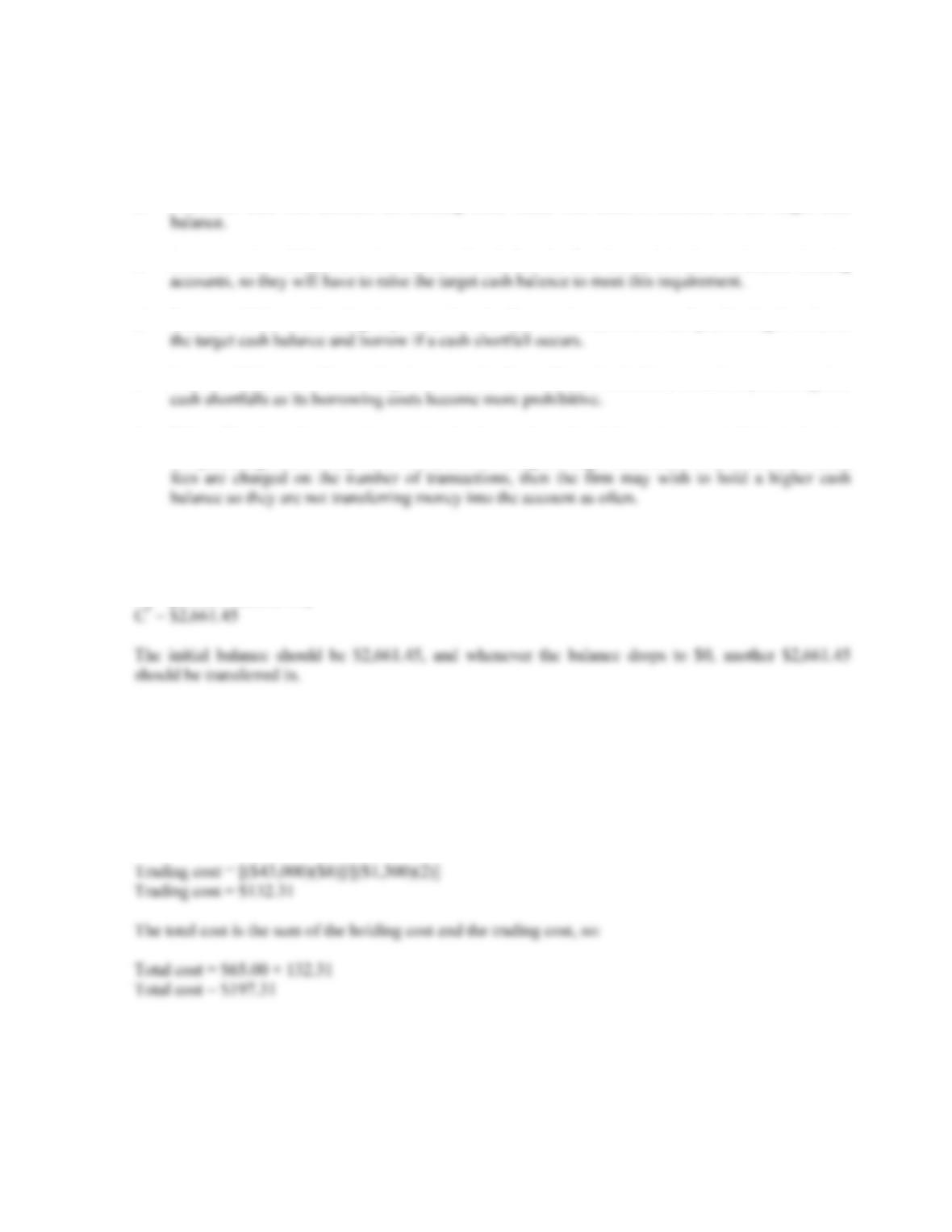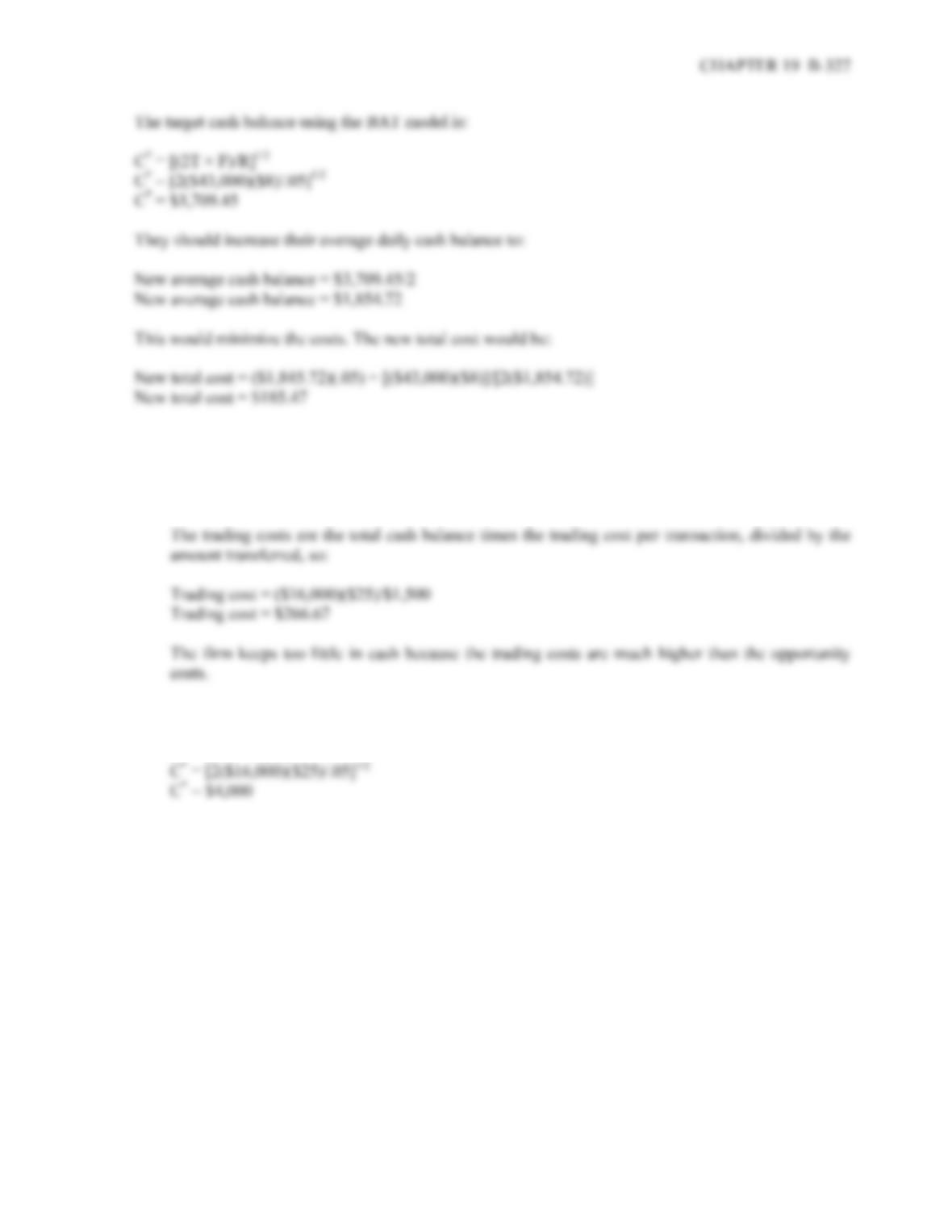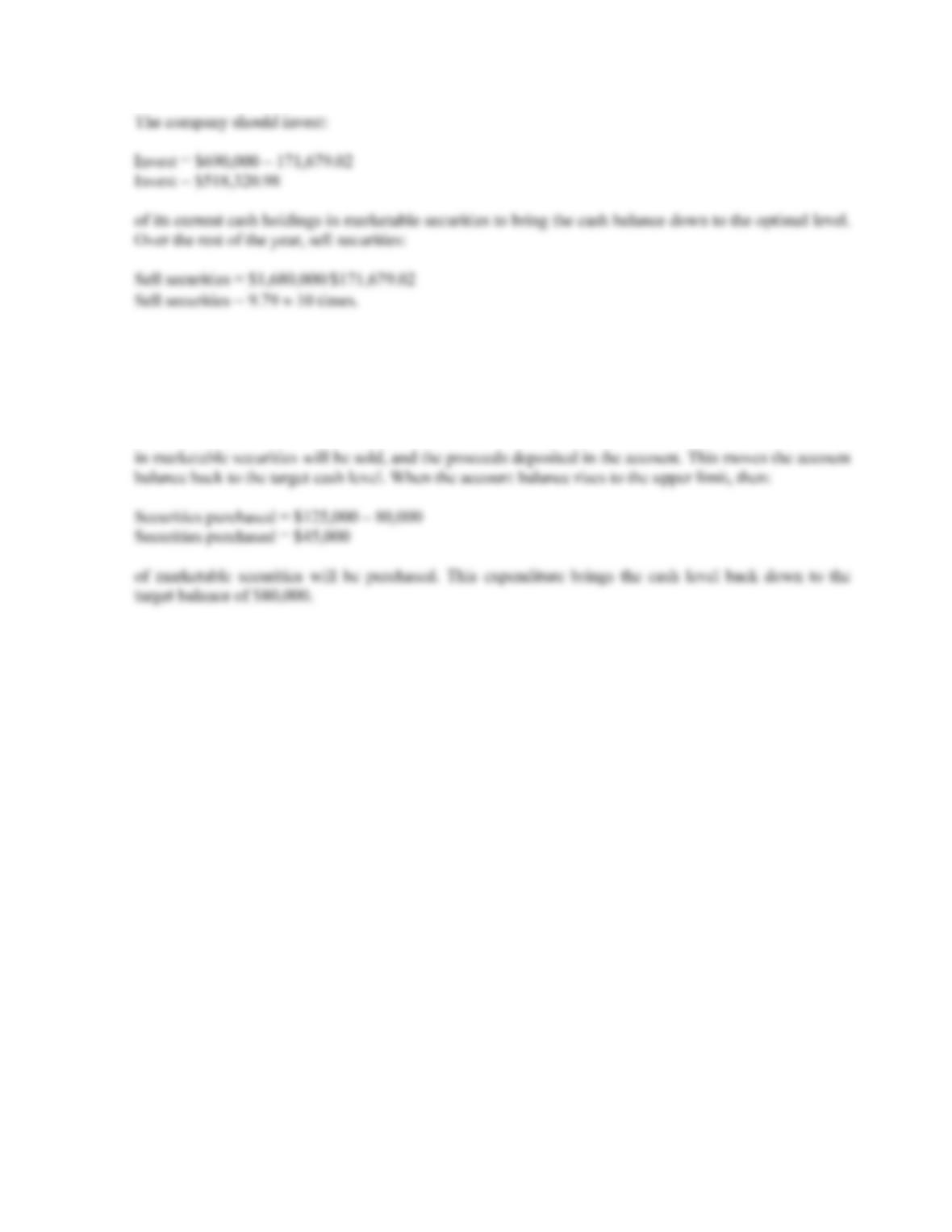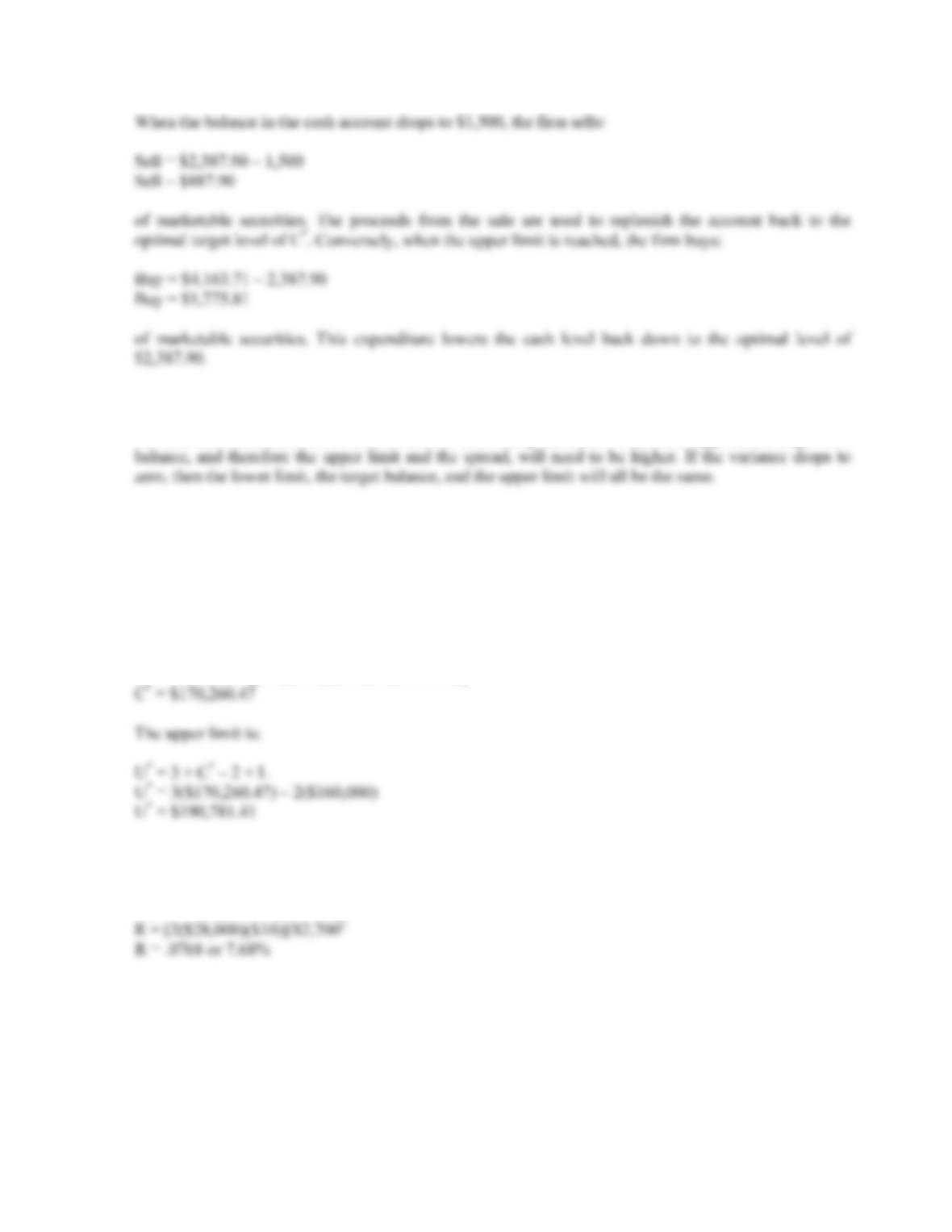Type
Quiz
Book Title
Fundamentals of Corporate Finance Standard Edition 9th Edition
ISBN 13
978-0073382395

### 978-0073382395 Chapter 19 Appendix

April 3, 2019B-326 SOLUTIONS
APPENDIX 19A
1. a. Decrease. This will lower the trading costs, which will cause a decrease in the target cash balance.
b. Decrease. This will increase the holding cost, which will cause a decrease in the target cash
c. Increase. This will increase the amount of cash that the firm has to hold in non-interest bearing
d. Decrease. If the credit rating improves, then the firm can borrow more easily, allowing it to lower
e. Increase. If the cost of borrowing increases, the firm will need to hold more cash to protect against
f. Either. This depends somewhat on what the fees apply to, but if direct fees are established, then the
compensating balance may be lowered, thus lowering the target cash balance. If, on the other hand,
2. The target cash balance using the BAT model is:
C
* = [(2T × F)/R]1/2
C
* = [2(\$8,500)(\$25)/.06]1/2
3. The holding cost is the average daily cash balance times the interest rate, so:
Holding cost = (\$1,300)(.05)
Holding cost = \$65.00
The trading costs are the total cash needed times the replenishing costs, divided by the average daily
balance times two, so:4. a. The opportunity costs are the amount transferred times the interest rate, divided by two, so:
Opportunity cost = (\$1,500)(.05)/2
Opportunity cost = \$37.50
b. The target cash balance using the BAT model is:
C
* = [(2T × F)/R]1/2
5. The total cash needed is the cash shortage per month times twelve months, so:
Total cash = 12(\$140,000)
Total cash = \$1,680,000
The target cash balance using the BAT model is:
C
* = [(2T × F)/R]1/2
C
* = [2(\$1,680,000)(\$500)/.057]1/2
C
* = \$171,679.02B-328 SOLUTIONS
6. The lower limit is the minimum balance allowed in the account, and the upper limit is the maximum
balance allowed in the account. When the account balance drops to the lower limit:
Securities sold = \$80,000 – 43,000
Securities sold = \$37,000
7. The target cash balance using the Miller-Orr model is:
C
* = L + (3/4 × F × 2 / R]1/3
C
* = \$1,500 + [3/4(\$40)(\$70)2/.00021]1/3
C
* = \$2,387.90
The upper limit is:
U
* = 3 × C* – 2 × L
U
* = 3(\$2,387.90) – 2(\$1,500)
U
* = \$4,163.71CHAPTER 19 B-329
8. As variance increases, the upper limit and the spread will increase, while the lower limit remains
unchanged. The lower limit does not change because it is an exogenous variable set by management. As
the variance increases, however, the amount of uncertainty increases. When this happens, the target cash
9. The average daily interest rate is:
Daily rate = 1.071/365 – 1
Daily rate = .000185 or .0185% per day
The target cash balance using the Miller-Orr model is:
C
* = L + (3/4 × F × 2 / R]1/3
C
* = \$160,000 + [3/4(\$300)(\$890,000)/.000185]1/3
10. Using the BAT model and solving for R, we get:
C
* = [(2T × F)/R]1/2
\$2,700 = [2(\$28,000)(\$10)/R]1/2